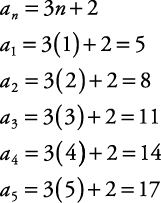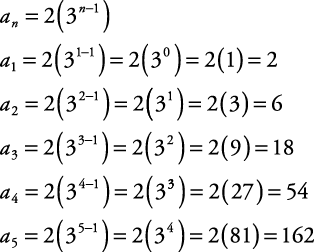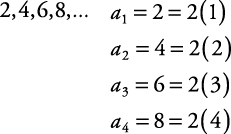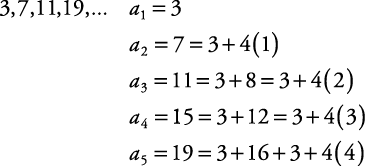## Definition and Examples of Sequences

A sequence is an ordered list of numbers .The three dots mean to continue forward in the pattern established. Each number in the sequence is called a term. In the sequence 1, 3, 5, 7, 9, …, 1 is the first term, 3 is the second term, 5 is the third term, and so on. The notation a 1, a 2, a 3,… a n is used to denote the different terms in a sequence.

The expression a n is referred to as the general or nth term of the sequence.

##### Example 1

Write the first five terms of a sequence described by the general term a n = 3 n + 2.Therefore, the first five terms are 5, 8, 11, 14, and 17.

##### Example 2

Write the first five terms of a n = 2(3 n – 1 ).Therefore, the first five terms are 2, 6, 18, 54, and 162.

##### Example 3

Find an expression for the nth term of each sequence.

1. 2, 4, 6, 8, …

2. 10, 50, 250, 1250, …

3. 3, 7, 11, 15, 19, …

1.Based on this pattern, a n = 2 n.

1.Based on this pattern, a n = 2(5 n ).

1.Based on this pattern,